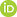Full Text:<1702>

Summary:<1509>

CLC number: TN911.7; O29

On-line Access: 2016-02-02

Revision Accepted: 2016-01-06

Crosschecked: 2016-01-14

Cited: 4

Clicked: 9488

Citations:  Bibtex RefMan EndNote GB/T7714ORCID:

Wei Liu

http://orcid.org/0000-0003-2968-2888

### -   Go to

Article info.
 Frontiers of Information Technology & Electronic Engineering  2016 Vol.17 No.2 P.83-95 http://doi.org/10.1631/FITEE.1500334Properties of a general quaternion-valued gradient operator and its applications to signal processing

 Author(s):  Meng-di Jiang, Yi Li, Wei Liu Affiliation(s):  1Department of Electronic and Electrical Engineering, University of Sheffield, Sheffield S1 3JD, UK; more Corresponding email(s):   w.liu@sheffield.ac.uk Key Words:  Quaternion, Gradient operator, Signal processing, Least mean square (LMS) algorithm, Nonlinear adaptive filtering, Adaptive beamforming Share this article to： More |Next Article >>>

Meng-di Jiang, Yi Li, Wei Liu. Properties of a general quaternion-valued gradient operator and its applications to signal processing[J]. Frontiers of Information Technology & Electronic Engineering, 2016, 17(2): 83-95.

@article{title="Properties of a general quaternion-valued gradient operator and its applications to signal processing",
author="Meng-di Jiang, Yi Li, Wei Liu",
journal="Frontiers of Information Technology & Electronic Engineering",
volume="17",
number="2",
pages="83-95",
year="2016",
publisher="Zhejiang University Press & Springer",
doi="10.1631/FITEE.1500334"
}

%0 Journal Article
%T Properties of a general quaternion-valued gradient operator and its applications to signal processing
%A Meng-di Jiang
%A Yi Li
%A Wei Liu
%J Frontiers of Information Technology & Electronic Engineering
%V 17
%N 2
%P 83-95
%@ 2095-9184
%D 2016
%I Zhejiang University Press & Springer
%DOI 10.1631/FITEE.1500334

TY - JOUR
T1 - Properties of a general quaternion-valued gradient operator and its applications to signal processing
A1 - Meng-di Jiang
A1 - Yi Li
A1 - Wei Liu
J0 - Frontiers of Information Technology & Electronic Engineering
VL - 17
IS - 2
SP - 83
EP - 95
%@ 2095-9184
Y1 - 2016
PB - Zhejiang University Press & Springer
ER -
DOI - 10.1631/FITEE.1500334

Abstract:
The gradients of a quaternion-valued function are often required for quaternionic signal processing algorithms. The HR gradient operator provides a viable framework and has found a number of applications. However, the applications so far have been limited to mainly real-valued quaternion functions and linear quaternion-valued functions. To generalize the operator to nonlinear quaternion functions, we define a restricted version of the HR operator, which comes in two versions, the left and the right ones. We then present a detailed analysis of the properties of the operators, including several different product rules and chain rules. Using the new rules, we derive explicit expressions for the derivatives of a class of regular nonlinear quaternion-valued functions, and prove that the restricted HR gradients are consistent with the gradients in the real domain. As an application, the derivation of the least mean square algorithm and a nonlinear adaptive algorithm is provided. Simulation results based on vector sensor arrays are presented as an example to demonstrate the effectiveness of the quaternion-valued signal model and the derived signal processing algorithm.

### Reference

Barthelemy, Q., Larue, A., Mars, J.I., 2014. About QLMS derivations. IEEE Signal Process. Lett., 21(2):240-243.Brandwood, D.H., 1983. A complex gradient operator and its application in adaptive array theory. IEE Proc. F, 130(1):11-16.Compton, R.T., 1981. On the performance of a polarization sensitive adaptive array. IEEE Trans. Antenn. Propag., 29(5):718-725.Ell, T.A., Sangwine, S.J., 2007. Quaternion involutions and anti-involutions. Comput. Math. Appl., 53(1):137-143.Ell, T.A., Le Bihan, N., Sangwine, S.J., 2014. Quaternion Fourier Transforms for Signal and Image Processing. Wiley, UK.

Gentili, G., Struppa, D.C., 2007. A new theory of regular functions of a quaternionic variable. Adv. Math., 216(1):279-301.Hawes, M., Liu, W., 2015. Design of fixed beamformers based on vector-sensor arrays. Int. J. Antenn. Propag., 2015:181937.1-181937.9.Jiang, M.D., Li, Y., Liu, W., 2014a. Properties and applications of a restricted HR gradient operator. arXiv:1407.5178.

Jiang, M.D., Liu, W., Li, Y., 2014b. A general quaternion-valued gradient operator and its applications to computational fluid dynamics and adaptive beamforming. Proc. 19th Int. Conf. on Digital Signal Processing, p.821-826.Jiang, M.D., Liu, W., Li, Y., 2014c. A zero-attracting quaternion-valued least mean square algorithm for sparse system identification. Proc. 9th Int. Symp. on Communication Systems, Networks and Digital Signal Processing, p.596-599.Le Bihan, N., Mars, J., 2004. Singular value decomposition of quaternion matrices: a new tool for vector-sensor signal processing. Signal Process., 84(7):1177-1199.Le Bihan, N., Miron, S., Mars, J.I., 2007. MUSIC algorithm for vector-sensors array using biquaternions. IEEE Trans. Signal Process., 55(9):4523-4533.Li, J., Compton, R.T., 1991. Angle and polarization estimation using ESPRIT with a polarization sensitive array. IEEE Trans. Antenn. Propag., 39:1376-1383.Liu, H., Zhou, Y.L., Gu, Z.P., 2014. Inertial measurement unit-camera calibration based on incomplete inertial sensor information. J. Zhejiang Univ.-Sci. C (Comput. & Electron.), 15(11):999-1008.Liu, W., 2014. Antenna array signal processing for a quaternion-valued wireless communication system. Proc. IEEE Benjamin Franklin Symp. on Microwave and Antenna Sub-systems, arXiv:1504.02921.

Liu, W., Weiss, S., 2010. Wideband Beamforming: Concepts and Techniques. Wiley, UK.

Mandic, D.P., Jahanchahi, C., Took, C.C., 2011. A quaternion gradient operator and its applications. IEEE Signal Process. Lett., 18(1):47-50.Miron, S., Le Bihan, N., Mars, J.I., 2006. Quaternion-MUSIC for vector-sensor array processing. IEEE Trans. Signal Process., 54(4):1218-1229.Parfieniuk, M., Petrovsky, A., 2010. Inherently lossless structures for eight- and six-channel linear-phase paraunitary filter banks based on quaternion multipliers. Signal Process., 90(6):1755-1767.Pei, S.C., Cheng, C.M., 1999. Color image processing by using binary quaternion-moment-preserving thresholding technique. IEEE Trans. Image Process., 8(5):614-628.Roberts, M.K., Jayabalan, R., 2015. An improved low-complexity sum-product decoding algorithm for low-density parity-check codes. Front. Inform. Technol. Electron. Eng., 16(6):511-518.Sangwine, S.J., Ell, T.A., 2000. The discrete Fourier transform of a colour image. Proc. Image Processing II: Mathematical Methods, Algorithms and Applications, p.430-441.

Talebi, S.P., Mandic, D.P., 2015. A quaternion frequency estimator for three-phase power systems. Proc. IEEE Int. Conf. on Acoustics, Speech and Signal Processing, p.3956-3960.Talebi, S.P., Xu, D.P., Kuh, A., et al., 2014. A quaternion least mean phase adaptive estimator. Proc. IEEE Int. Conf. on Acoustics, Speech and Signal Processing, p.6419-6423.Tao, J.W., 2013. Performance analysis for interference and noise canceller based on hypercomplex and spatio-temporal-polarisation processes. IET Radar Sonar Navig., 7(3):277-286.Tao, J.W., Chang, W.X., 2014. Adaptive beamforming based on complex quaternion processes. Math. Prob. Eng., 2014:291249.1-291249.10.Zhang, X.R., Liu, W., Xu, Y.G., et al., 2014. Quaternion-valued robust adaptive beamformer for electromagnetic vector-sensor arrays with worst-case constraint. Signal Process., 104:274-283.